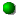# \$B%=!<%[!

C/1996 X5 = C/2002 S5 ( SOHO )###\$B%/%i%O%H72\$N7OE}?^(B\$B@8\$-;D\$C\$F\$\$\$kWB@1(B\$B>CLG\$7\$?WB@1(BC/2004 A3C/1999 M3 = C/2004 L10C/1999 N6 = C/2004 J4 = C/2009 L8C/2009 Y10342P/2000 O3 = P/2005 W4 = P/2011 E1 = P/2016 N5C/2001 Q7C/2001 R8C/2002 Q8 = C/2008 E4C/1996 X3 = C/2002 N2C/1996 X3 = C/2002 S7 = C/2008 N4C/1996 X5 = C/2002 S5C/2002 S4 = C/2008 R7C/2002 S11 = C/2008 G6

###\$B2s5"5-O?(B\$BH/8+(B\$B=P8=(B\$B4QB,\$5\$l\$J\$+\$C\$?2s5"(B\$BH/8+A0\$N=P8=(B\$BH/8+A0\$N4QB,\$5\$l\$J\$+\$C\$?2s5"(B\$B>-Mh\$N2s5"(BC/2002 S5 2002/09/19C/1996 X5 1996/12/06##### C/1996 X5 \$B\$H(B C/2002 S5 \$B\$NF1Dj\$O(BM.P.E.C. 2009-T27\$B\$K7G:\\$5\$l\$?\$b\$N!#(B